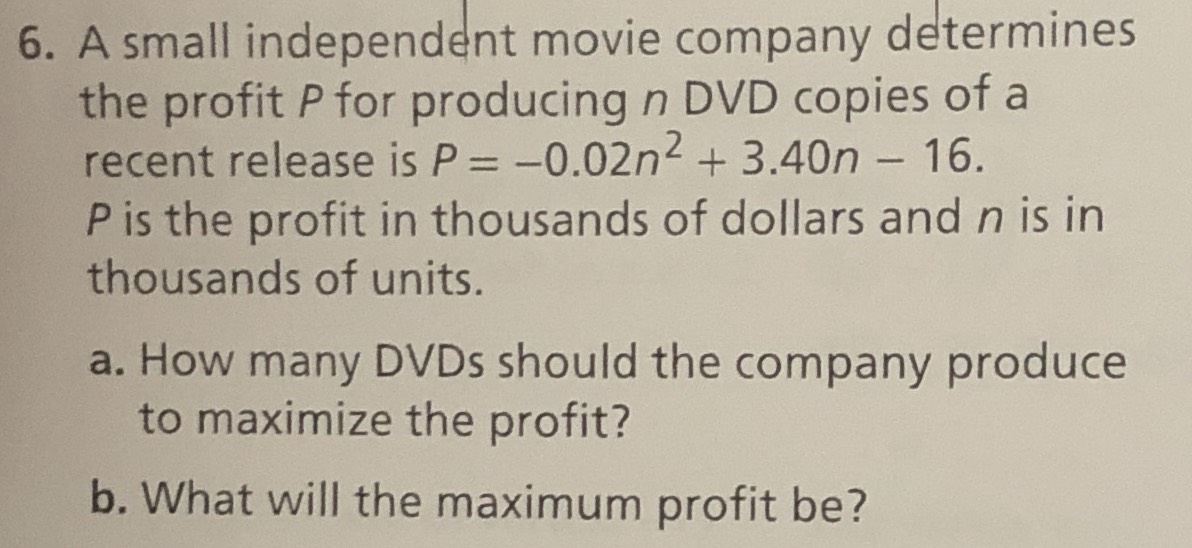### ¿Todavía tienes preguntas de matemáticas?

Pregunte a nuestros tutores expertos
Algebra
Pregunta6. A small independent movie company determines the profit $$P$$ for producing $$n$$ DVD copies of a recent release is $$P = - 0.02 n ^ { 2 } + 3.40 n - 16$$ .

$$P$$ is the profit in thousands of dollars and $$n$$ is in thousands of units.

a. How many DVDs should the company produce to maximize the profit?

b. What will the maximum profit be?

a) to find the maximum, differentiate P w.r.t.n then set it to zero

dP/dn = -0.04n+3.40

-0.04n+3.40=0

0.04n=3.40

n= 85 units

b) maximum profit

P(85)= -0.02(85)^2 +3.40(85)-16 = \$128.5

Solución
View full explanation on CameraMath App.